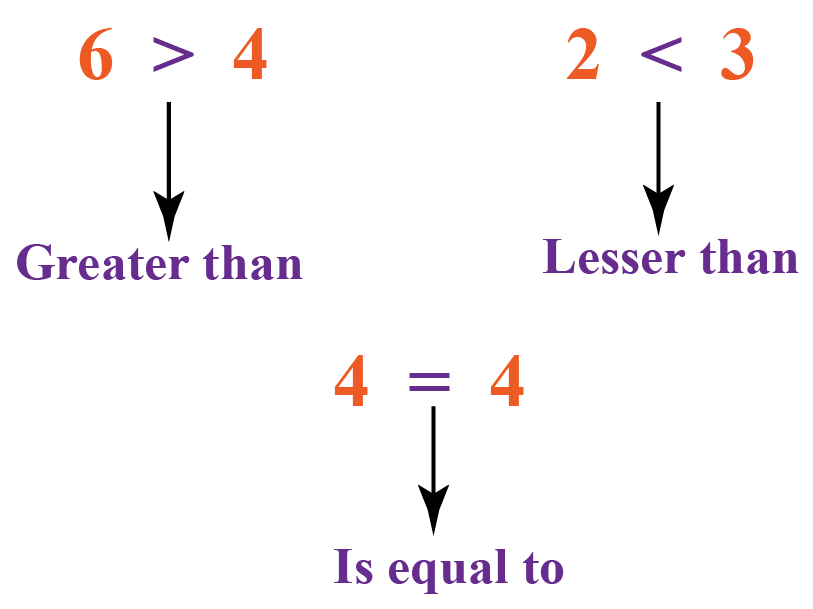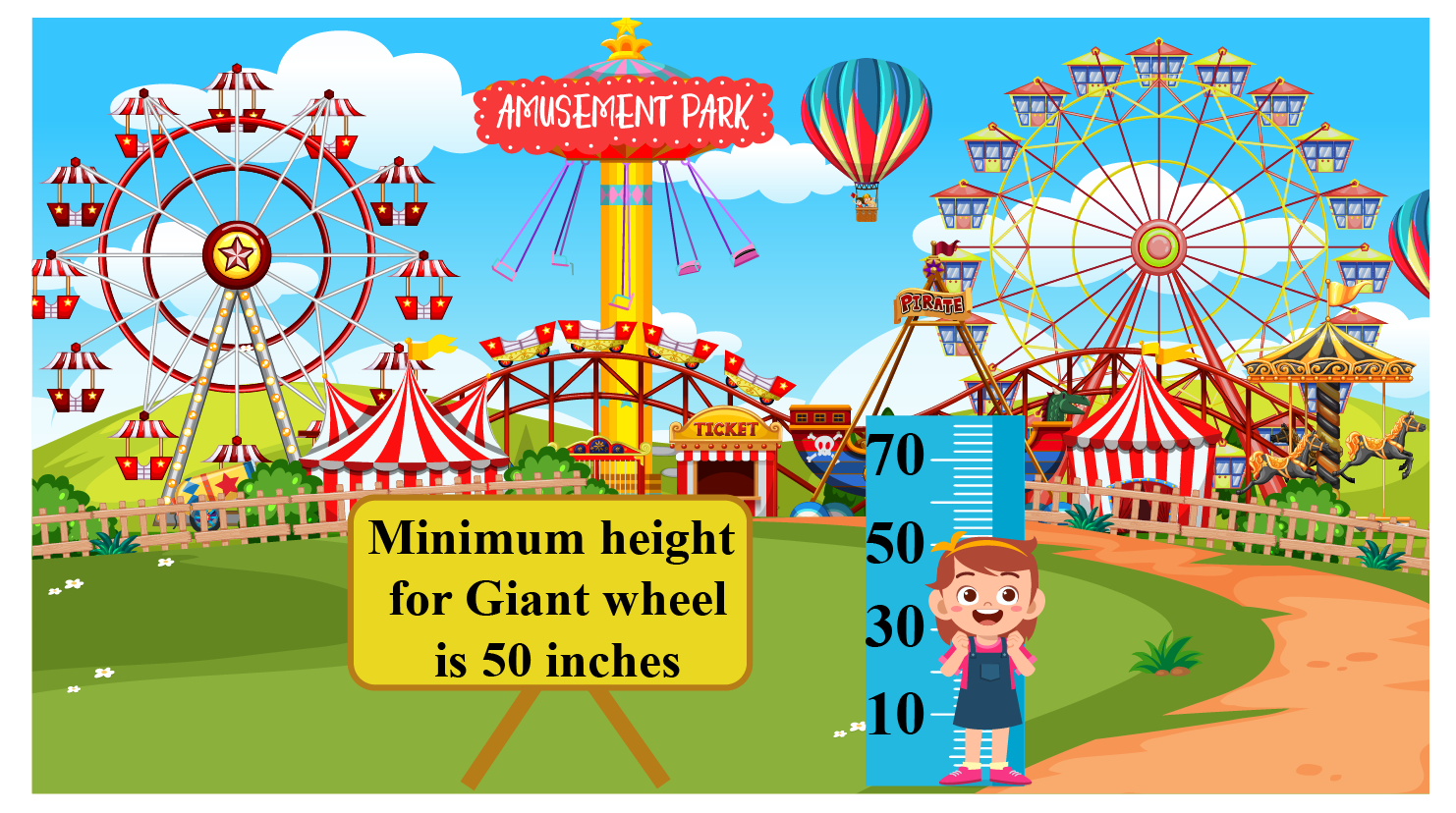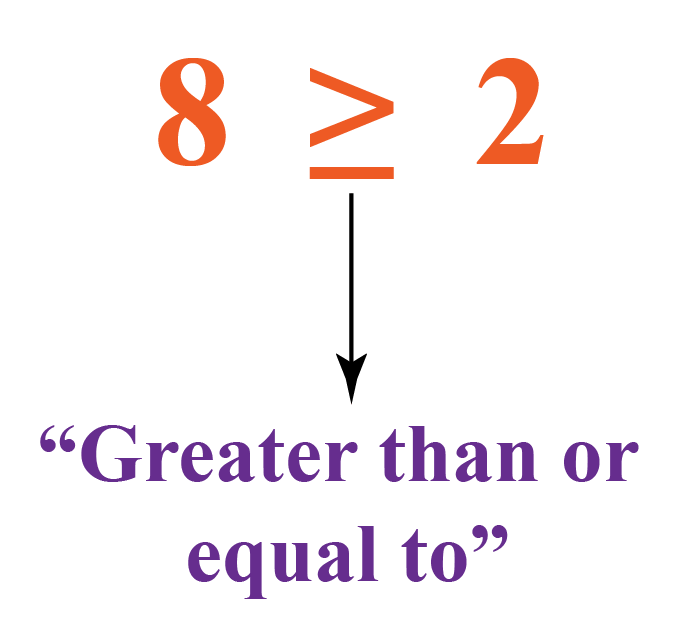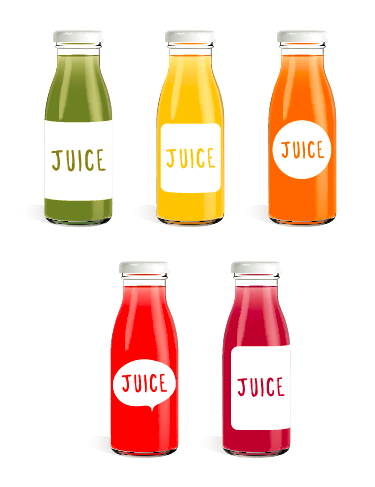# Greater than or Equal to Sign

Greater than or Equal to Sign

## Table of Contents

 1 Introduction to "Greater Than or Equal To" 2 Greater Than or Equal To: Math Definition 3 Greater Than or Equal To: Math Examples 4 Activity on Greater Than or Equal To 5 Greater Than and Lesser Than Symbols 6 Tips and Tricks 6 Solved-Examples 7 Practice Questions 8 Challenging Questions 9 Maths Olympiad Sample Papers 10 Frequently Asked Questions (FAQs)

We at Cuemath believe that Math is a life skill. Our Math Experts focus on the “Why” behind the “What.” Students can explore from a huge range of interactive worksheets, visuals, simulations, practice tests, and more to understand a concept in depth.

Book a FREE trial class today! and experience Cuemath’s LIVE Online Class with your child.

## Introduction to "Greater Than or Equal To"

We already know symbols for "greater than $$(>$$)", "lesser than $$(<)$$" and "is equal to $$(=)$$"

We know that the:

• "greater than" symbol is used to show that one quantity is greater than the other quantity.
• "lesser than" symbol is used to show that one quantity is lesser than the other quantity.
• "is equal to" symbol is used to show that two quantities are equal.What if we have to say that some quantity is either greater than or equal to a number?

Let us consider this example.

Linda wanted to enjoy a ride in a giant wheel at the amusement park.

At the entrance, she saw a board with instructions.

The instructions stated that the minimum height of the person riding the giant wheel should be $$50$$ inches.She asked her parents about the meaning of the word "minimum".

Her mother explained that only people with height "greater than or equal to" $$50$$ inches would be allowed in the giant wheel.

We come across situations like this in our daily lives where the usage of "greater than or equal to" is a must.

## Greater Than or Equal To: Math Definition

"Greater than or equal to", as the suggests, means something is either greater than or equal to another thing.

"Greater than or equal to" is represented by the symbol " $$\geq$$".

This symbol is nothing but the "greater than" symbol with a sleeping line under it.

We know that $$8$$ is greater than $$2$$

This obviously means that $$8$$ is greater than or equal to $$2$$

Hence, we represent it as $$8 \geq 2$$, using the "greater than or equal to" symbol in between $$8$$ and $$2$$However, using the "greater than or equal to" symbol in case of numbers doesn't make much sense because either ">" or "=" is sufficient to represent the relationship.

So when do we normally use "greater than or equal to"?

Let us learn about this next.

## Greater Than or Equal To: Math Examples

Here is an example for you to understand this concept better.

For a school to participate in an olympiad exam, the number of students from each class should be a minimum of $$10$$

This means that if there are less than $$10$$ students participating from any one of the classes, none of the students of that class can take the olympiad exam.If $$x$$ represents the number of students participating from a class, then "$$x$$ should be greater than or equal to $$10$$"

This is represented by:

$${x \geq 10}$$

• $$x \geq 100$$ means the value of $$x$$ should be greater than or equal to $$100$$. Here are some other Math examples for "Greater than or equal to".
• $$a \geq -2$$ means the value of $$a$$ should be greater than or equal to $$-2$$

## Activity on Greater Than or Equal To

You can try the following activity to understand what is greater than or equal to.

## Greater Than and Lesser Than Symbols

Here are some comparison symbols and their examples along with their meanings.

Symbol Example  Meaning
Greater than or equal to, $$\geq$$

$$x \geq 2$$

The value of $$x$$ is greater than or equal to $$2$$

$$2 \geq x \geq -1$$

The value of $$x$$ should between $$-1$$ and $$2$$ inclusive of both values.
Lesser than or equal to, $$\leq$$

$$x \leq 7$$

The value of $$x$$ is lesser than or equal to $$7$$

$$-5 \leq x \leq 3$$

The value of $$x$$ should between $$-5$$ and $$3$$ inclusive of both values.

You can find the symbols for "greater than" and "lesser than" by clicking here.Tips and Tricks
1. The words "minimum", "at least", "greater than or equal to", etc. correspond to the sign $$\mathbf{\geq }$$.
2. The words "maximum", "at most", "lesser than or equal to", etc. correspond to the sign $$\mathbf{\leq }$$.

Help your child score higher with Cuemath’s proprietary FREE Diagnostic Test. Get access to detailed reports, customised learning plans and a FREE counselling session. Attempt the test now.

## Solved Examples

 Example 1

For a person to be elected as a president, he should be a minimum of $$35$$ years old.If $$A$$ represents the person's age, write an inequality representing this information.

Solution:

It is given that the person's age $$(A)$$ should be minimum $$35$$

This means that $$A$$ should be either greater than or equal to $$35$$

Hence, this is represented by the inequality,

 $${A \geq 35}$$
 Example 2

Find the numbers that belong to the following set.

$\{x \in N/ x \geq 3\}$

Solution:

We know that $$N$$ is the set of natural numbers.

The given set is:

$\{x \in N/ x \geq 3\}$

This means that we have to find all the natural numbers that are greater than or equal to $$3$$

Since the set of natural numbers is infinite, the numbers that belong to the given set are:

 $${3,4,5,6,...}$$
 Example 3

Charles had $$18$$ chocolates which he was going to distribute to his friends on his birthday.

Since the box was open, he lost some chocolates on the way.If $$x$$ is the number of chocolates that Charles currently has, write an inequality that represents this situation.

Solution:

Number of chocolates Charles had initially = $$18$$

Since he lost some chocolates, the number of chocolates with him currently is less than $$18$$

$x < 18 \,\,\,\,\, \rightarrow (1)$

We know that the number of chocolates should be a non-nagative quantity. Hence,

$x \geq 0$

This inequality can also be written as:

$0 \leq x \,\,\,\,\, \rightarrow (2)$

From the inequalities (1) and (2),

 $${0 \leq x < 18}$$
 Example 4

A fruit shop owner sells a glass of juice for $$\ 3$$

He has a target of earning revenues of at least $$350$$ a day.If $$x$$ is the number of glasses of juice that he sells in a day, write an inequality representing this situation.

Solution:

Cost of each glass of juice = $$\ 3$$

Number of glasses of juice he sells in a day = $$x$$

Hence, the total cost of $$x$$ the juices he sells in a day = $$3x$$

We know that the revenue should be "at least" $$350$$ a day.

This means the total revenue should be "greater than or equal to" $$350$$ a day.

This is represented by the inequality:

 $${ 3x \geq 350}$$

CLUEless in Math? Check out how CUEMATH Teachers will explain Greater than or equal to to your kid using interactive simulations & worksheets so they never have to memorise anything in Math again!

Explore Cuemath Live, Interactive & Personalised Online Classes to make your kid a Math Expert. Book a FREE trial class today!

## Practice Questions

Here are a few activities for you to practice. Select/Type your answer and click the "Check Answer" button to see the result.Challenging Questions
1. Olivia scored $$89, 62,$$ and $$75$$ in her first three math exams.
Write an inequality that shows the marks she should get in her fourth exam to get an average of at least $$80$$ on her four exams.
2. The cost to publish a book is $$\ 1000$$ with an additional cost of $$\ 5$$ per book.
The book sells for $$\12$$ each.
Write an inequality to show the profit to be generated.

## Maths Olympiad Sample Papers

IMO (International Maths Olympiad) is a competitive exam in Mathematics conducted annually for school students. It encourages children to develop their math solving skills from a competition perspective.

You can download the FREE grade-wise sample papers from below:

To know more about the Maths Olympiad you can click here

## 1. How do you write greater than or equal to?

To show the greater than symbol, write the "greater than" symbol along with a sleeping line below it.

$$\geq$$ is the greater than or equal to symbol.

## 2. What does $$\geq$$ mean (or) What is the Math definition of greater than or equal to?

"Greater than or equal to", as the name suggests, means something is either greater than or equal to some other thing.

"Greater than or equal to" is represented by the symbol " $$\geq$$".

For example, $$x \geq -2$$ means the value of $$x$$ should be greater than or equal to $$-2$$.

You can learn more from the "Greater or equal to: Math Definition" section of this page.

## 3. What does a greater than or equal to sign look like?

A greater than or equal to symbol is shown as the "greater than" symbol along with a sleeping line below it.

$$\geq$$ is the greater than or equal to symbol.

More Important Topics
Numbers
Algebra
Geometry
Measurement
Money
Data
Trigonometry
Calculus